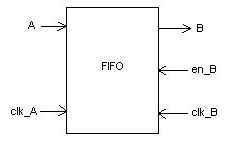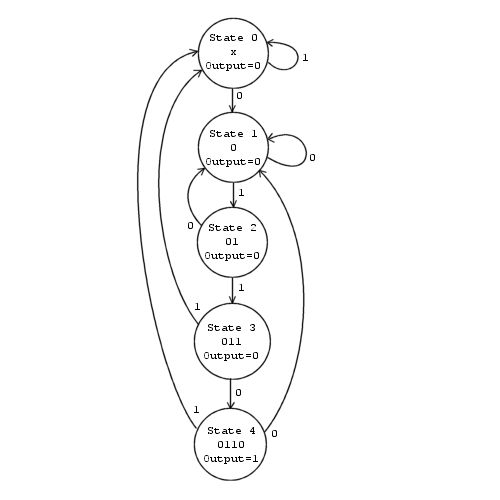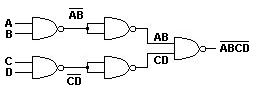# Electrical EngineeringTechnical InterviewQuestions/Review

I've included some sample interview questions below to be used as a review for Electrical Engineering. These are typical of the types of questions asked as part of a hardware design/verification position interview.

If anyone has any unique questions (and answers) that you'd like to see included, please email them to me at tom<at>grumpytom.com Also, please let me know if something is unclear or just plain wrong!

These questions have been broken into the following categories:

• Verilog
• Vera
• Programming
• General Engineering

## Questions

```Verilog Question 1
Q: What is the difference between a Verilog task and a Verilog function?

```

```Verilog Question 2
Q: Given the following Verilog code, what value of "a" is displayed?

always @(clk) begin
a  = 0;
a <= 1;
\$display(a);
end

```

```Verilog Question 3
Q: Given the following snipet of Verilog code,
draw out the waveforms for "clk" and "a".

always @(clk) begin
a = 0;
#5 a = 1;
end

```

```Verilog Question 4
Q: What is the difference between the following two lines of Verilog code?

#5 a = b;
a = #5 b;

```

```Verilog Question 5
Q: Write the Verilog code to provide a divide-by-3 clock from the standard clock.

```

```Verilog Question 6
Q: What is the difference between:

c = foo ? a : b;

and

if (foo) c = a;
else c = b;

```

```Verilog Question 7
Q: Using the given, draw the waveforms for the following
versions of a (each version is separate, i.e. not in the same run):

reg clk;
reg a;

always #10 clk = ~clk;

(1) always @(clk) a = # 5 clk;
(2) always @(clk) a = #10 clk;
(3) always @(clk) a = #15 clk;

Now, change a to wire, and draw for:

(4) assign #5  a = clk;
(5) assign #10 a = clk;
(6) assign #15 a = clk;

```

```Vera Question 1
Q: What is the difference between a Vera task and a Verilog task?

```

```Vera Question 2
Q: What is the difference between running the following snipet of code
on Verilog vs Vera?

fork {
#10;
}

cnt = 0;
for (i = 0; i < 50; i++) {
cnt++;
}
}

```

```Programming Question 1
Q: Given \$a = "5,-3,7,0,-5,12";

Write a program to find the lowest number in the string.

```

```Programming Question 2
Q: Write the code to sort an array of integers.

```

```Programming Question 3
Q: Write the code to find the factorial of an integer.
Use a recursive subroutine.

```

```Programming Question 4
Q: In C, explain the difference between the & operator and
the * operator.

```

```Programming Question 5
Q: Write a function to determine whether a string is a palindrome (same
forward as reverse, such as "radar" or "mom").

```

```Programming Question 6
Q: Write a function to output a diamond shape according to the given (odd) input.

Examples:  Input is 5    Input is 7

*             *
***           ***
*****         *****
***         *******
*           *****
***
*

```

```General Question 1
Q: Given the following FIFO and rules, how deep does the FIFO need to be to
prevent underflowing or overflowing?RULES:
1) frequency(clk_A) = frequency(clk_B) / 4
2) period(en_B) = period(clk_A) * 100
3) duty_cycle(en_B) = 25%

```

```General Question 2
Q: Draw the state diagram to output a "1" for one cycle
if the sequence "0110" shows up (the leading 0s cannot be
used in more than one sequence).

```

```General Question 3
Q: Explain the differences between "Direct Mapped", "Fully Associative",
and "Set Associative" caches.

```

```General Question 4
Q: Design a four-input NAND gate using only two-input NAND gates.

```

```General Question 5
Q: Draw the state diagram for a circuit that outputs a "1" if the aggregate serial
binary input is divisible by 5.  For instance, if the input stream is 1, 0, 1, we
output a "1" (since 101 is 5). If we then get a "0", the aggregate total is 10, so
we output another "1" (and so on).

```

```Verilog Answer 1
Q: What is the difference between a Verilog task and a Verilog function?

A:

The following rules distinguish tasks from functions:

A function shall execute in one simulation time unit;
a task can contain time-controlling statements.

A function cannot enable a task;

A function shall have at least one input type argument
and shall not have an output or inout type argument;
a task can have zero or more arguments of any type.

A function shall return a single value;
a task shall not return a value.

(Back)

```

```Verilog Answer 2
Q: Given the following Verilog code, what value of "a" is displayed?

always @(clk) begin
a  = 0;
a <= 1;
\$display(a);
end

A:

This is a tricky one!  Verilog scheduling semantics basically imply a
four-level deep queue for the current simulation time:

1: Active Events                (blocking statements)
2: Inactive Events              (#0 delays, etc)
4: Monitor Events               (\$display, \$monitor, etc).

Since the "a = 0" is an active event, it is scheduled into the 1st "queue".
The "a <= 1" is a non-blocking event, so it's placed into the 3rd queue.
Finally, the display statement is placed into the 4th queue.

Only events in the active queue are completed this sim cycle, so the "a = 0"
happens, and then the display shows a = 0.  If we were to look at the value of
a in the next sim cycle, it would show 1.

(Back)

```

```Verilog Answer 3
Q: Given the following snipet of Verilog code,
draw out the waveforms for clk and a

always @(clk) begin
a = 0;
#5 a = 1;
end

A:

10      30      50      70      90     110     130
___     ___     ___     ___     ___     ___     ___
clk ___|   |___|   |___|   |___|   |___|   |___|   |___|   |___

a   ___________________________________________________________

This obviously is not what we wanted, so to get closer, you could use
"always @ (posedge clk)" instead, and you'd get

10      30      50      70      90     110     130
___     ___     ___     ___     ___     ___     ___
clk ___|   |___|   |___|   |___|   |___|   |___|   |___|   |___

___                     ___
a   _______________________|   |___________________|   |_______

(Back)

```

```Verilog Answer 4
Q: What is the difference between the following two lines of Verilog code?

#5 a = b;
a = #5 b;

A:

#5 a = b;  Wait five time units before doing the action for "a = b;".
The value assigned to a will be the value of b 5 time units hence.

a = #5 b;  The value of b is calculated and stored in an internal temp register.
After five time units, assign this stored value to a.

(Back)

```

```Verilog Answer 6
Q: What is the difference between:

c = foo ? a : b;

and

if (foo) c = a;
else c = b;

A:

The ? merges answers if the condition is "x", so for instance if foo = 1'bx, a = 'b10, and b = 'b11,
you'd get c = 'b1x.

On the other hand, if treats Xs or Zs as FALSE, so you'd always get c = b.

(Back)

```

```Verilog Answer 7

Q: Using the given, draw the waveforms for the following
versions of a (each version is separate, i.e. not in the same run):

reg clk;
reg a;

always #10 clk = ~clk;

(1) always @(clk) a = #5  clk;
(2) always @(clk) a = #10 clk;
(3) always @(clk) a = #15 clk;

Now, change a to wire, and draw for:

(4) assign #5  a = clk;
(5) assign #10 a = clk;
(6) assign #15 a = clk;

A:

10      30      50      70      90     110     130
___     ___     ___     ___     ___     ___     ___
clk ___|   |___|   |___|   |___|   |___|   |___|   |___|   |___

___     ___     ___     ___     ___     ___     ___
(1)a ____|   |___|   |___|   |___|   |___|   |___|   |___|   |_

___     ___     ___     ___     ___     ___     ___
(2)a ______|   |___|   |___|   |___|   |___|   |___|   |___|

(3)a __________________________________________________________

Since the #delay cancels future events when it activates, any delay
over the actual 1/2 period time of the clk flatlines...

With changing a to a wire and using assign, we
just accomplish the same thing...

10      30      50      70      90     110     130
___     ___     ___     ___     ___     ___     ___
clk ___|   |___|   |___|   |___|   |___|   |___|   |___|   |___

___     ___     ___     ___     ___     ___     ___
(4)a ____|   |___|   |___|   |___|   |___|   |___|   |___|   |_

___     ___     ___     ___     ___     ___     ___
(5)a ______|   |___|   |___|   |___|   |___|   |___|   |___|

(6)a __________________________________________________________

(Back)

```

```Vera Answer 1
Q: What is the difference between a Vera task and a Verilog task?

A:

(Back)

```

```Vera Answer 2
Q: What is the difference between running the following snipet of code
on Verilog vs Vera?

fork {
#10;
}

cnt = 0;
for (i = 0; i < 50; i++) {
cnt++;
}
}

A:

(Back)

```

```Programming Answer 1
Q: Given \$a = "5,-3,7,0,-5,12";

Write a program to find the lowest number in the string.

A:

// BEGIN PERL SNIPET

\$a = "5,-5,-1,0,12,-3";
(@temp) = split (/,/, \$a);
\$lowest = \$temp;

for (\$i=0; \$i<6; \$i++) {
if (\$temp[\$i] < \$lowest) { \$lowest = \$temp[\$i]; }
}

print "Lowest value found was: \$lowest\n";

// END PERL SNIPET

NOTE: You could also replace the for loop with this:

foreach \$value (@temp) {
if (\$value < \$lowest) { \$lowest = \$value; }
}

(Back)

```

```Programming Answer 2
Q: Write the code to sort an array of integers.

A:

/* BEGIN C SNIPET */

void bubblesort (int x[], int lim) {
int i, j, temp;

for (i = 0; i < lim; i++) {
for (j = 0; j < lim-1-i; j++) {

if (x[j] > x[j+1]) {
temp = x[j];
x[j] = x[j+1];
x[j+1] = temp;

} /* end if         */
}    /* end for j      */
}       /* end for i      */
}          /* end bubblesort */

/* END C SNIPET */

Some optimizations that can be made are that a single-element array does
not need to be sorted; therefore, the "for i" loop only needs to go from
0 to lim-1.  Next, if at some point during the iterations, we go through
the entire array WITHOUT performing a swap, the complete array has been
sorted, and we do not need to continue.  We can watch for this by adding
a variable to keep track of whether we have performed a swap on this
iteration.

(Back)

```

```Programming Answer 3
Q: Write the code for finding the factorial of a passed integer.
Use a recursive subroutine.

A:

// BEGIN PERL SNIPET

sub factorial {
my \$y = shift;
if ( \$y > 1 ) {
return \$y * &factorial( \$y - 1 );
} else {
return 1;
}
}

// END PERL SNIPET

(Back)

```

```Programming Answer 4
Q: In C, explain the difference between the & operator and
the * operator.

A:

& is the address operator, and it creates pointer values.
* is the indirection operator, and it dereferences pointers
to access the object pointed to.

Example:
In the following example, the pointer ip is assigned the
address of variable i (&i).  After that assignment,
the expression *ip refers to the same object denoted by i:

int i, j, *ip;
ip = &i;
i = 22;
j = *ip;    /* j now has the value 22 */
*ip = 17;   /* i now has the value 17 */

(Back)

```

```Programming Answer 5
Q: Write a function to determine whether a string is a palindrome (same
forward as reverse, such as "radar" or "mom").

A:

/* BEGIN C SNIPET */

#include <string.h>

void is_palindrome ( char *in_str ) {
char *tmp_str;
int  i, length;

length = strlen ( *in_str );
for ( i = 0; i < length; i++ ) {
*tmp_str[length-i-1] = *in_str[i];
}
if ( 0 == strcmp ( *tmp_str, *in_str ) ) printf ("String is a palindrome");
else printf ("String is not a palindrome");
}

/* END C SNIPET */

(Back)

```

```Programming Answer 6
Q: Write a function to output a diamond shape according to the given (odd) input.

Examples:  Input is 5    Input is 7

*             *
***           ***
*****         *****
***         *******
*           *****
***
*

A:

### BEGIN PERL SNIPET ###

for (\$i = 1; \$i <= ((\$input * 2) - 1); \$i += 2) {
if (\$i <= \$input) {
\$stars = \$i;
\$spaces = (\$input - \$stars) / 2;
while (\$spaces--) { print " "; }
while (\$stars--) { print "*"; }
} else {
\$spaces = (\$i - \$input) / 2;
\$stars = \$input - (\$spaces * 2);
while (\$spaces--) { print " "; }
while (\$stars--) { print "*"; }
}
print "\n";
}
### END PERL SNIPET ###

(Back)

```

```General Answer 1
Q: Given the following FIFO and rules, how deep does the FIFO need to be to
prevent underflowing or overflowing?RULES:
1) frequency(clk_A) = frequency(clk_B) / 4
2) period(en_B) = period(clk_A) * 100
3) duty_cycle(en_B) = 25%

A:

Assume clk_B = 100MHz (10ns)

From (1), clk_A = 25MHz (40ns)

From (2), period(en_B) = 40ns * 100 = 4000ns, but we only output for 1000ns,
due to (3), so 3000ns of the enable we are doing no output work.

Therefore, FIFO size = 3000ns/40ns = 75 entries.

(Back)

```

```General Answer 2
Q: Draw the state diagram to output a "1" for one cycle
if the sequence "0110" shows up (the leading 0s cannot be
used in more than one sequence).

A:(Back)

```

```General Answer 3
Q: Explain the differences between "Direct Mapped", "Fully Associative",
and "Set Associative" caches.

A:

If each block has only one place it can appear in the cache, the cache
is said to be direct mapped.  The mapping is usually (block-frame address)
modulo (number of blocks in cache).

If a block can be placed anywhere in the cache, the cache is said to be
fully associative.

If a block can be placed in a restricted set of places in the cache, the cache
is said to be set associative.  A set is a group of two or more
blocks in the cache.  A block is first mapped onto a set, and then the block
can be placed anywhere within the set.  The set is usually chosen by bit
selection; that is, (block-frame address) modulo (number of sets in cache).
If there are n blocks in a set, the cache placement is
called n-way set associative.

(Back)

```

```General Answer 4
Q: Design a four-input NAND gate using only two-input NAND gates.

A:

Basically, you can tie the inputs of a NAND gate together to get an inverter, so...(Back)

```

```General Answer 5
Q: Draw the state diagram for a circuit that outputs a "1" if the aggregate serial
binary input is divisible by 5.  For instance, if the input stream is 1, 0, 1, we
output a "1" (since 101 is 5). If we then get a "0", the aggregate total is 10, so
we output another "1" (and so on).

A:

We don't need to keep track of the entire string of numbers - if something
is divisible by 5, it doesn't matter if it's 250 or 0, so we can just reset to 0.
So we really only need to keep track of "0" through "4".(Back)

```